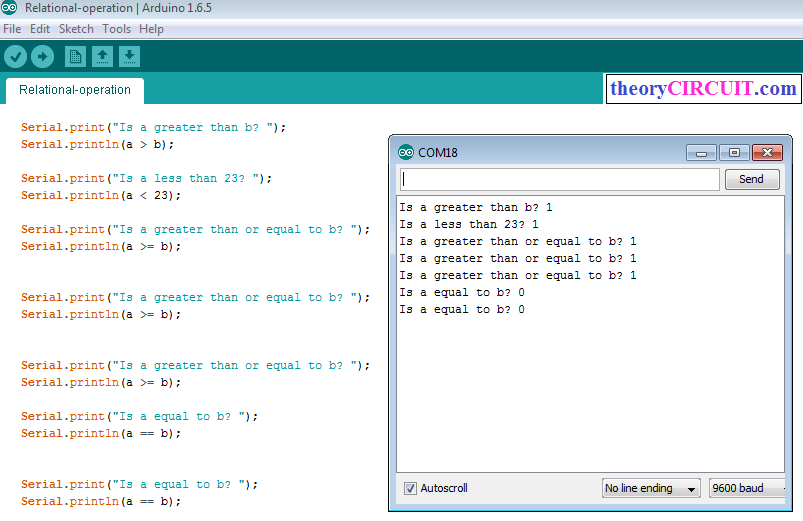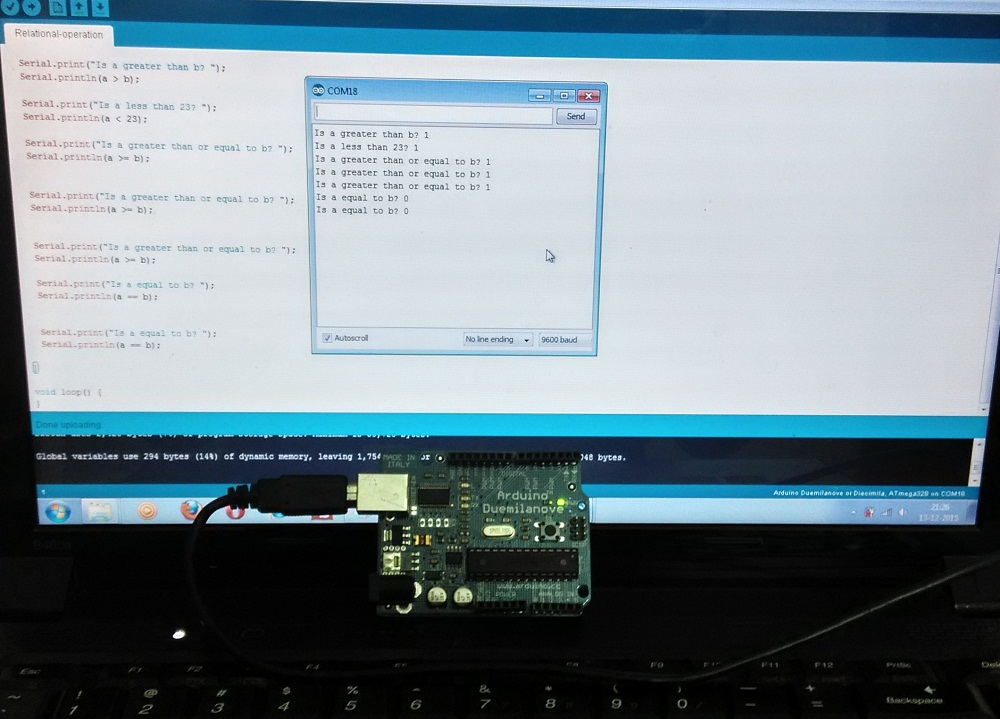# Arduino Relational functions

The arduino can do Relational functions between two numbers, simple example and syntax of relational operations are shown.The arduino performs

#### Less than or Equal to (<=);     Equal to (==);     Not Equal to (!=);

For all these Relational operation the result will be true or false, by the way the serial monitor shows it as 1(true) and 0(false), by using conditional statements we can do control over the digital output pins.

### Arduino Code

```void setup() {
int a = 22;
int b = 10;

Serial.begin(9600);

Serial.print("Is a greater than b? ");
Serial.println(a > b);

Serial.print("Is a less than 23? ");
Serial.println(a < 23);

Serial.print("Is a greater than or equal to b? ");
Serial.println(a >= b);

Serial.print("Is a greater than or equal to b? ");
Serial.println(a >= b);

Serial.print("Is a greater than or equal to b? ");
Serial.println(a >= b);

Serial.print("Is a equal to b? ");
Serial.println(a == b);

Serial.print("Is a equal to b? ");
Serial.println(a == b);

}

void loop() {
}

```

### Screen shot